Download Presentation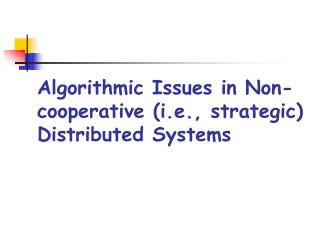Algorithmic Issues in Non-cooperative (i.e., strategic) Distributed Systems

# Algorithmic Issues in Non-cooperative (i.e., strategic) Distributed Systems - PowerPoint PPT Presentation

Algorithmic Issues in Non-cooperative (i.e., strategic) Distributed Systems Two Research Traditions Theory of Algorithms: computational issues What can be feasibly computed? How much does it take to compute a solution? Which is the quality of a computed solution?I am the owner, or an agent authorized to act on behalf of the owner, of the copyrighted work described.
Download Presentation## Algorithmic Issues in Non-cooperative (i.e., strategic) Distributed Systems

An Image/Link below is provided (as is) to download presentation

Download Policy: Content on the Website is provided to you AS IS for your information and personal use and may not be sold / licensed / shared on other websites without getting consent from its author.While downloading, if for some reason you are not able to download a presentation, the publisher may have deleted the file from their server.

- - - - - - - - - - - - - - - - - - - - - - - - - - E N D - - - - - - - - - - - - - - - - - - - - - - - - - -
Presentation Transcript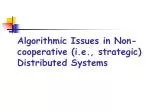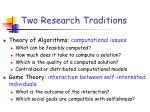• Theory of Algorithms: computational issues
• What can be feasibly computed?
• How much does it take to compute a solution?
• Which is the quality of a computed solution?
• Centralized or distributed computational models
• Game Theory: interaction between self-interested individuals
• What is the outcome of the interaction?
• Which social goals are compatible with selfishness?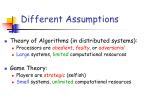Different Assumptions
• Theory of Algorithms (in distributed systems):
• Processors are obedient, faulty, or adversarial
• Large systems, limited computational resources
• Game Theory:
• Players are strategic(selfish)
• Small systems, unlimited computational resourcesThe Internet World
• Agents often autonomous (users)
• Users have their own individual goals
• Network components owned by providers
• Often involve “Internet” scales
• Massive systems
• Limited communication/computational resources

 Both strategic and computational issues!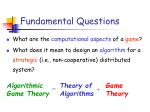Fundamental Questions
• What are the computational aspects of a game?
• What does it mean to design an algorithm for a strategic (i.e., non-cooperative) distributed system?

Algorithmic

Game Theory

Theory of Algorithms

Game Theory

=

+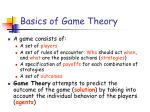Basics of Game Theory
• A game consists of:
• A set of players
• A set of rules of encounter: Who should act when, and what are the possible actions (strategies)
• A specification of payoffs for each combination of strategies
• A set of outcomes
• Game Theory attempts to predict the outcome of the game (solution) by taking into account the individual behavior of the players (agents)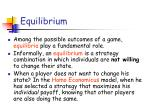Equilibrium
• Among the possible outcomes of a game, equilibria play a fundamental role.
• Informally, an equilibrium is a strategy combination in which individuals are not willing to change their state.
• When a player does not want to change his state? In the Homo Economicus model, when he has selected a strategy that maximizes his individual payoff, knowing that other players are also doing the same.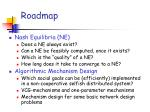• Nash Equilibria (NE)
• Does a NE always exist?
• Can a NE be feasibly computed, once it exists?
• Which is the “quality” of a NE?
• How long does it take to converge to a NE?
• Algorithmic Mechanism Design
• Which social goals can be (efficiently) implemented in a non-cooperative selfish distributed system?
• VCG-mechanisms and one-parameter mechanisms
• Mechanism design for some basic network design problemsFIRST PART:

(Nash)

Equilibria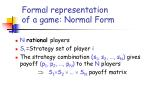Formal representation of a game: Normal Form
• Nrational players
• Si=Strategy set of player i
• The strategy combination (s1, s2, …, sN) gives payoff (p1, p2, …, pN) to the N players

 S1S2 …  SN payoff matrixTypes of games
• Cooperative versus non-cooperative
• Symmetric versus asymmetric
• Zero sum versus non-zero sum
• Simultaneous versus sequential
• Perfect information versus imperfect information
• One-shot versus repeatedA famous one-shot game: the Prisoner’s Dilemma

…the story of two strange and dangerous fellows…Prisoner I’s decision
• Prisoner I’s decision:
• If II chooses Don’t Implicate then it is best to Implicate
• If II chooses Implicate then it is best to Implicate
• It is best to Implicate for I, regardless of what II does: Dominant StrategyPrisoner II’s decision
• Prisoner II’s decision:
• If I chooses Don’t Implicatethen it is best to Implicate
• If I chooses Implicatethen it is best to Implicate
• It is best to Implicate for II, regardless of what I does: Dominant StrategyHence…
• It is best for both to implicate regardless of what the other one does
• Implicate is a Dominant Strategy for both
• (Implicate, Implicate) becomes the Dominant Strategy Equilibrium
• Note: If they might collude, then it’s beneficial for both to Not Implicate, but it’s not an equilibrium as both have incentive to deviateA network game

C, S: peering points

s1

two Internet Service Providers (ISP):

ISP1 e ISP2

t2

C

S

ISP1 wants to send traffic from s1 to t1

t1

ISP2 wants to send traffic from s2 to t2

(long) links have cost 1

(for ISP owning the link)

s2

Each ISPi can use two paths: the one passing through C o

the one passing through SA network game

C, S: peering points

s1

t2

Cost Matrix

C

S

t1

ISP2

s2

ISP1Dominant Strategy Equilibrium
• Dominant Strategy Equilibrium: is a strategy combination s*= (s1*, s2*, …, sN*), such that si* is a dominant strategy for each i, namely, for each s= (s1, s2, …, si , …, sN):

pi(s1, s2, …, si*, …, sN) ≥ pi(s1, s2, …, si, …, sN)

• Dominant Strategy is the best response to any strategy of other players
• It is good for agent as it needs not to deliberate about other agents’ strategies
• Of course, not all games (only very few in the practice!) have a dominant strategy equilibriumA more relaxed solution concept: Nash Equilibrium 
• Nash Equilibrium: is a strategy combination

s*= (s1*, s2*, …, sN*) such that for each i, si* is a best response to (s1*, …,si-1*,si+1*,…, sN*), namely, for any possible alternative strategy si

pi(s*) ≥ pi(s1*, s2*, …, si, …, sN*)Nash Equilibrium
• In a NE no agent can unilaterally deviate from its strategy given others’ strategies as fixed
• Agent has to deliberate about the strategies of the other agents
• If the game is played repeatedly and players converge to a solution, then it has to be a NE
• Dominant Strategy Equilibrium  Nash Equilibrium (but the converse is not true)• (Stadium, Stadium) is a NE: Best responses to each other
• (Cinema, Cinema) is a NE: Best responses to each other

 but they are not Dominant Strategy Equilibria … are we really sure they will eventually go out together????A similar game: routing congestion game

O

two traffic streams originated

at node O need to be routed to

the rest of the network

1 2

5 6

A

B

Costs without congestion:

c(O,A)=1 c(O,B)=2

network

Costs with congestion:

c(O,A)=5 c(O,B)=6

Each stream can use two paths: the one passing through A o

the one passing through BA similar game: routing congestion game

O

1 2

5 6

A

B

Cost Matrix

network

stream 2

stream

1A big game theoretic issue: the existence of a NE
• Unfortunately, for pure strategies games (as those seen so far), it is easy to see that we cannot have a general result of existence
• In other words, there may be no, one, or many NE, depending on the gameA conflictual game: Head or Tail
• Player I (row) prefers to do what Player II does, while Player II prefer to do the opposite of what Player I does!

 In any configuration, one of the players prefers to change his strategy, and so on and so forth…thus, there are no NE!On the existence of a NE (2)
• However, when a player can select his strategy stochastically by using a probability distribution over his set of possible strategies (mixed strategy), then the following general result holds:
• Theorem (Nash, 1951): Any game with a finite set of players and a finite set of strategies has a NE of mixed strategies (i.e., the expectedpayoff cannot be improved by changing unilaterally the selected probability distribution).
• Head or Tail game: if each player sets p(Head)=p(Tail)=1/2, then the expected payoff of each player is 0, and this is a NE, since no player can improve on this by choosing a different randomization!Three big computational issues
• Finding a NE, once it does exist
• Establishing the quality of a NE, as compared to a cooperative system, i.e., a system in which agents can cooperate (recall the Prisoner’s Dilemma)
• In a repeated game, establishing whether and in how many steps the system will eventually converge to a NE (recall the Battle of the Sex)On the computability of a NEfor pure strategies
• By definition, it is easy to see that an entry (p1,…,pN) of the payoff matrix is a NE if and only if pi is the maximum ith element of the row (p1,…,pi-1,{p(s):sSi},pi+1,…,pN), for each i=1,…,N.
• Notice that, with N players, an explicit (i.e., in normal form) representation of the payoff functions is exponential in N brute-force search for pure NE is then exponential in the number of players (even if it is still linear in the input size, but the normal form representation needs not be a minimal-space representation of the input!)

 Alternative cheaper methods are sought (we will see that for some categories of games of our interest, a NE can be found in poly-time w.r.t. to the number of players)On the quality of a NE
• How inefficient is a NE in comparison to an idealized situation in which the players would strive to collaborate selflessly with the common goal of maximizing the social welfare?
• Recall: in the Prisoner’s Dilemma game, the DSE  NE means a total of 8 years in jail for the players. However, if they would not implicate reciprocally, then they would stay a total of only 4 years in jail!The price of anarchy (PoA)
• Definition (Koutsopias & Papadimitriou, 1999): Given a game G and a social-choice minimization (resp., maximization) function f (i.e., the sum of all players’ payoffs), let S be the set of NE, and let OPT be the outcome of G optimizing f. Then, the Price of Anarchy (PoA) of G w.r.t. f is:
• Example: in the PD game, G(f)=8/4=2The price of stability (PoS)
• Definition (Schulz & Moses, 2003): Given a game G and a social-choice minimization (resp., maximization) function f (i.e., the sum of all players’ payoffs), let S be the set of NE, and let OPT be the outcome of G optimizing f. Then, the Price of Stability (PoS) of G w.r.t. f is:Some remarks
• PoA and PoS are
•  1 for minimization problems
•  1 for maximization problems
• PoA and PoS are small when they are close to 1
• PoS is at least as close to 1 than PoA
• In a game with a unique equilibrium PoA=PoS
• PoA is similar to the concept of approximation ratio of a heuristic
• a bound on the PoS provides a significantly weaker guarantee than a bound on the PoA
• Why to study the PoS?
• sometimes a nontrivial bound is possible only for PoS
• PoS quantifies th necessary degradation in quality under the game-theoretic constraint of stabilityPollution game

There are n countries. Each country faces the choice of either passing legislation to control pollution or not.

Assume that pollution control has a cost of 3 for the country, but each country that pollutes adds 1 of all countries (in term of added health costs).

The cost of controlling pollution is 3.

...notice that the cost of controlling pollution

is considerably larger than the cost a country

pays for being socially irresponsible…Tragedy of commons

There are n players. Each player wants to send information along a shared channel of known maximum capacity 1. Player i’s strategy is to send xi units of flow along the channel, for some xi[0,1].

Each player would like to have a large fraction of the bandwidth but the quality of the channel deteriorates as the total assigned bandwidth increases. More precisely, if jxj exceeds 1, no player gets any benefit. Otherwise, the value of a player i is xi(1- jxj).